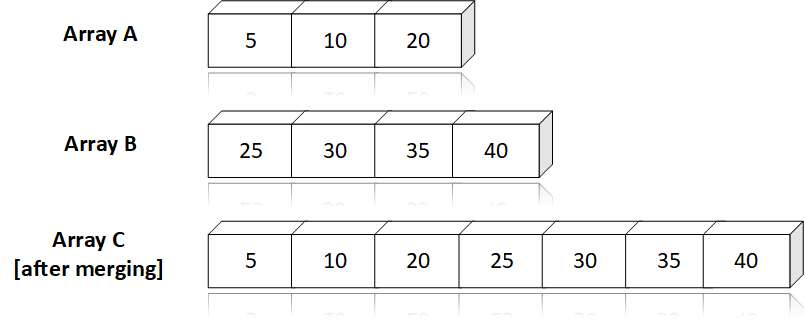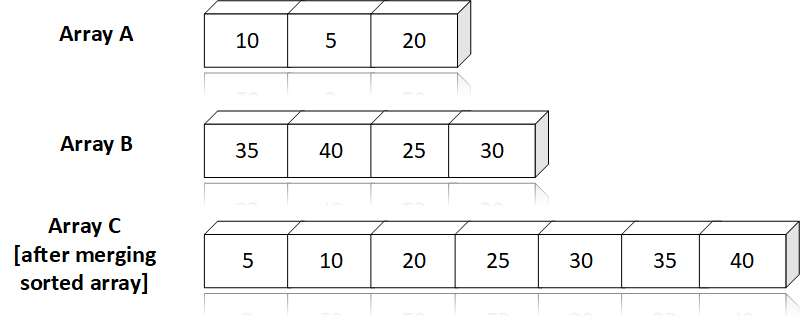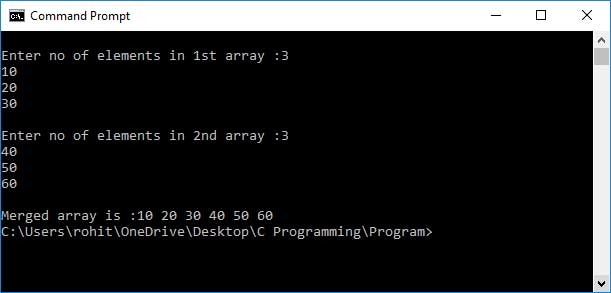# Array C Programing

Answer: Merging is the process of combining the two arrays into a single array. Merging can he done in two ways:

• Unsorted Array
• Sorted Array

Merging Unsorted Array: If the arrays are not sorted, we can combine them end to end i.e. we can first put the elements of first array into third array and then the elements of second array are placed after it in third array.

For Example:Merging Sorted Array: In many cases, we may have soiled arrays and our aim is to combine them in such way that the combined array is also in sorted order. One straight forward approach is to join them end to end and then sort the combined array, but this approach is not efficient and economical.

Therefore the best approach is to compare the elements of the given array, and based on this comparison, decide which element should go first to the third array.

For Example:Code:

``````
#include

int main() {
int arr1, arr2, res;
int i, j, k, n1, n2;

printf("\nEnter no of elements in 1st array :");
scanf("%d", &n1);
for (i = 0; i < n1; i++) {
scanf("%d", &arr1[i]);
}

printf("\nEnter no of elements in 2nd array :");
scanf("%d", &n2);
for (i = 0; i < n2; i++) {
scanf("%d", &arr2[i]);
}

i = 0;
j = 0;
k = 0;

// Merging starts
while (i < n1 && j < n2) {
if (arr1[i] <= arr2[j]) {
res[k] = arr1[i];
i++;
k++;
} else {
res[k] = arr2[j];
k++;
j++;
}
}

/* Some elements in array 'arr1' are still remaining
where as the array 'arr2' is exhausted */

while (i < n1) {
res[k] = arr1[i];
i++;
k++;
}

/* Some elements in array 'arr2' are still remaining
where as the array 'arr1' is exhausted */

while (j < n2) {
res[k] = arr2[j];
k++;
j++;
}

//Displaying elements of array 'res'
printf("\nMerged array is :");
for (i = 0; i < n1 + n2; i++)
printf("%d ", res[i]);

return (0);

}

``````

Output: